# RD Sharma Solutions for Class 8 Chapter 9 Linear Equation in One Variable Exercise 9.3

RD Sharma Solutions for Class 8 Maths Exercise 9.3 Chapter 9 is provided here. In Exercise 9.3, we shall discuss the concept of cross-multiplication method for solving equations. RD Sharma Solutions which is designed by our experts at BYJU’S to help students understand the concepts clearly. On regular practice, students can top their exams. Students can download the pdf easily from the links provided below.

## Download the pdf of RD Sharma for Class 8 Maths Exercise 9.3 Chapter 9 Linear Equation in One Variable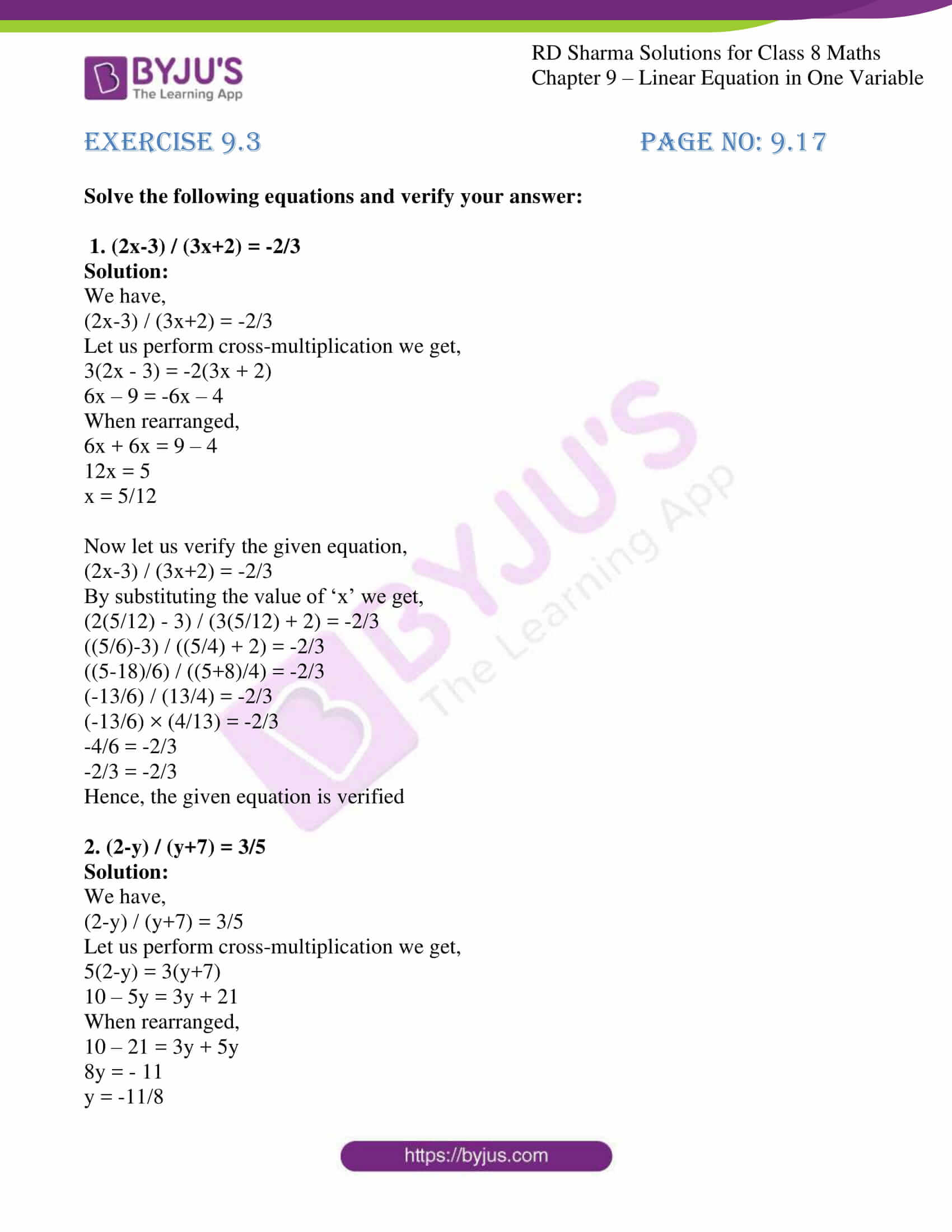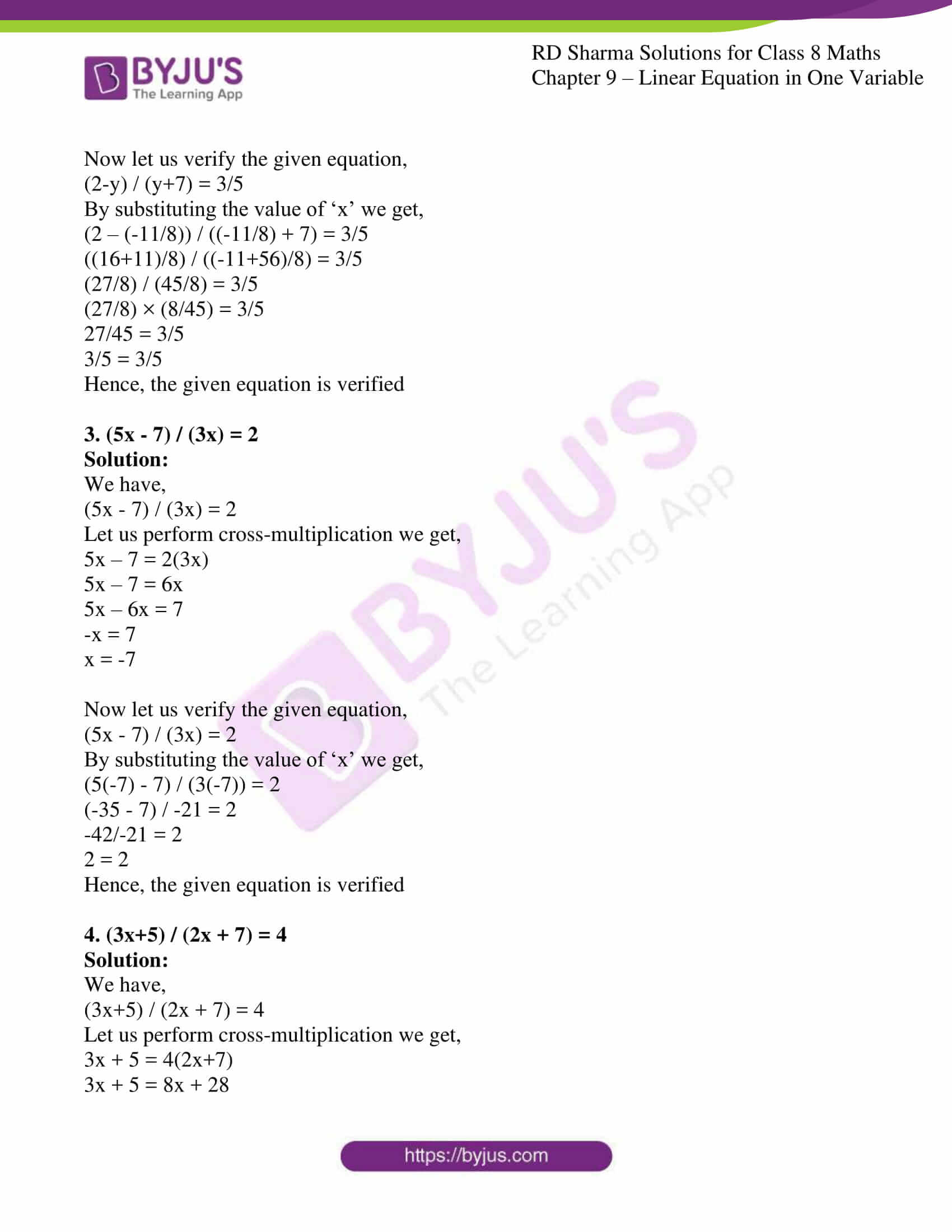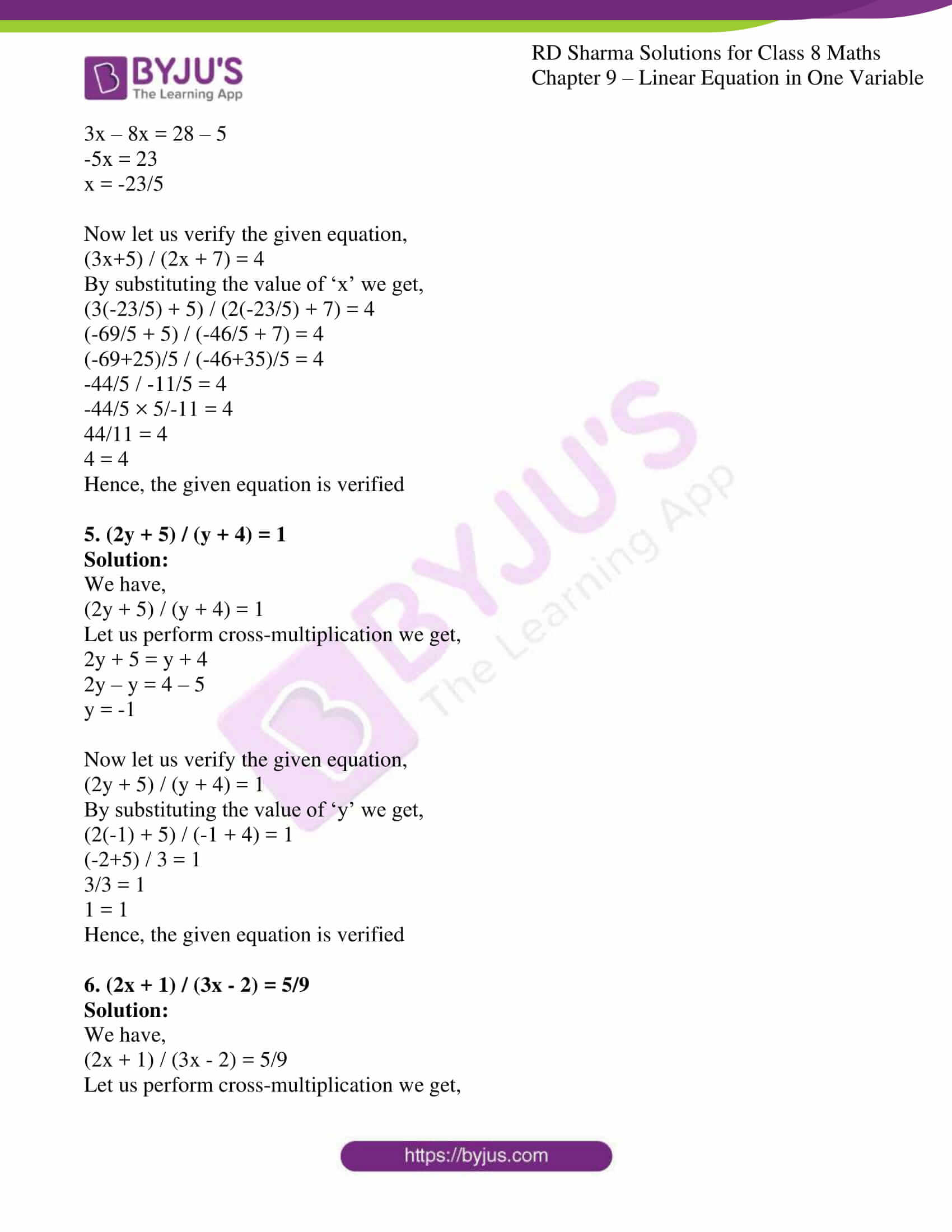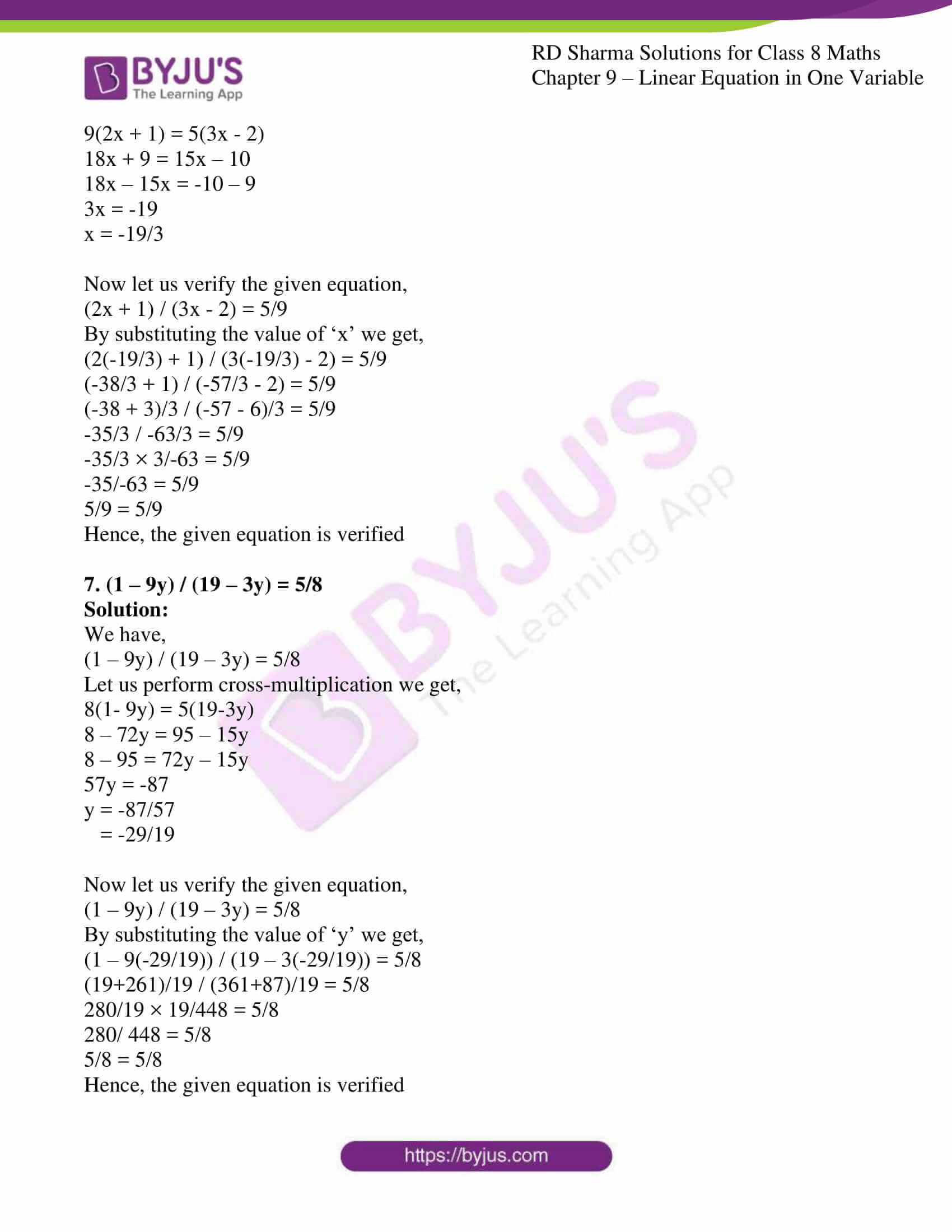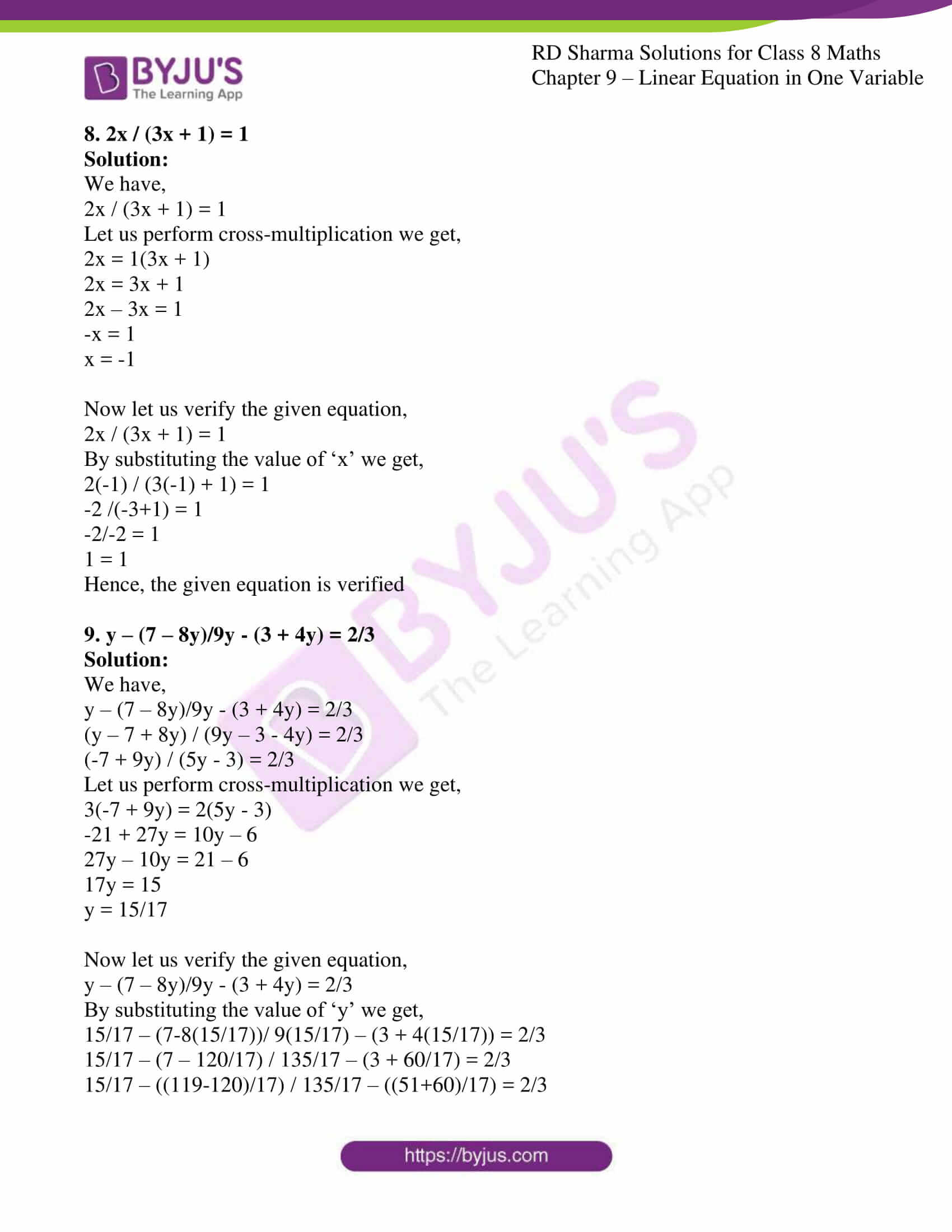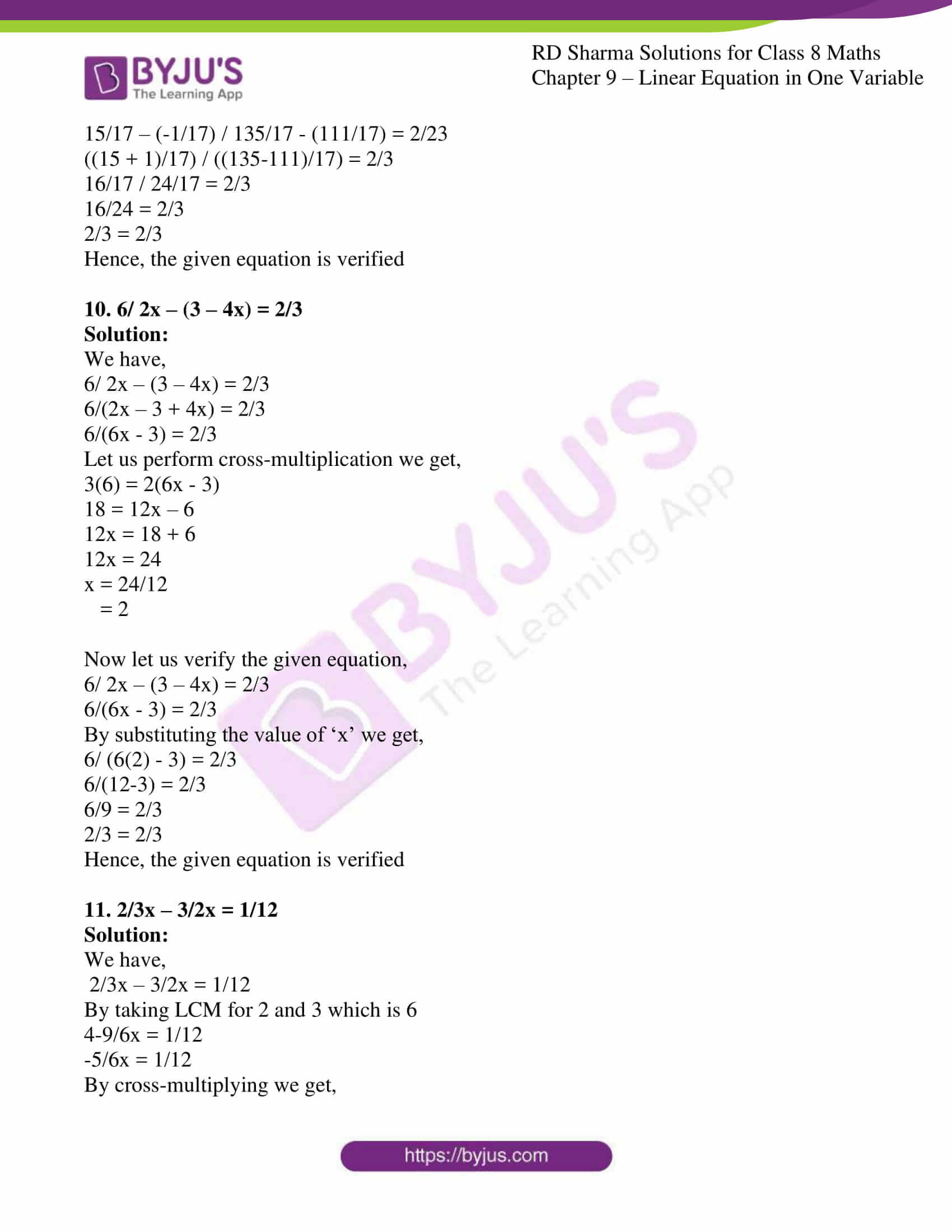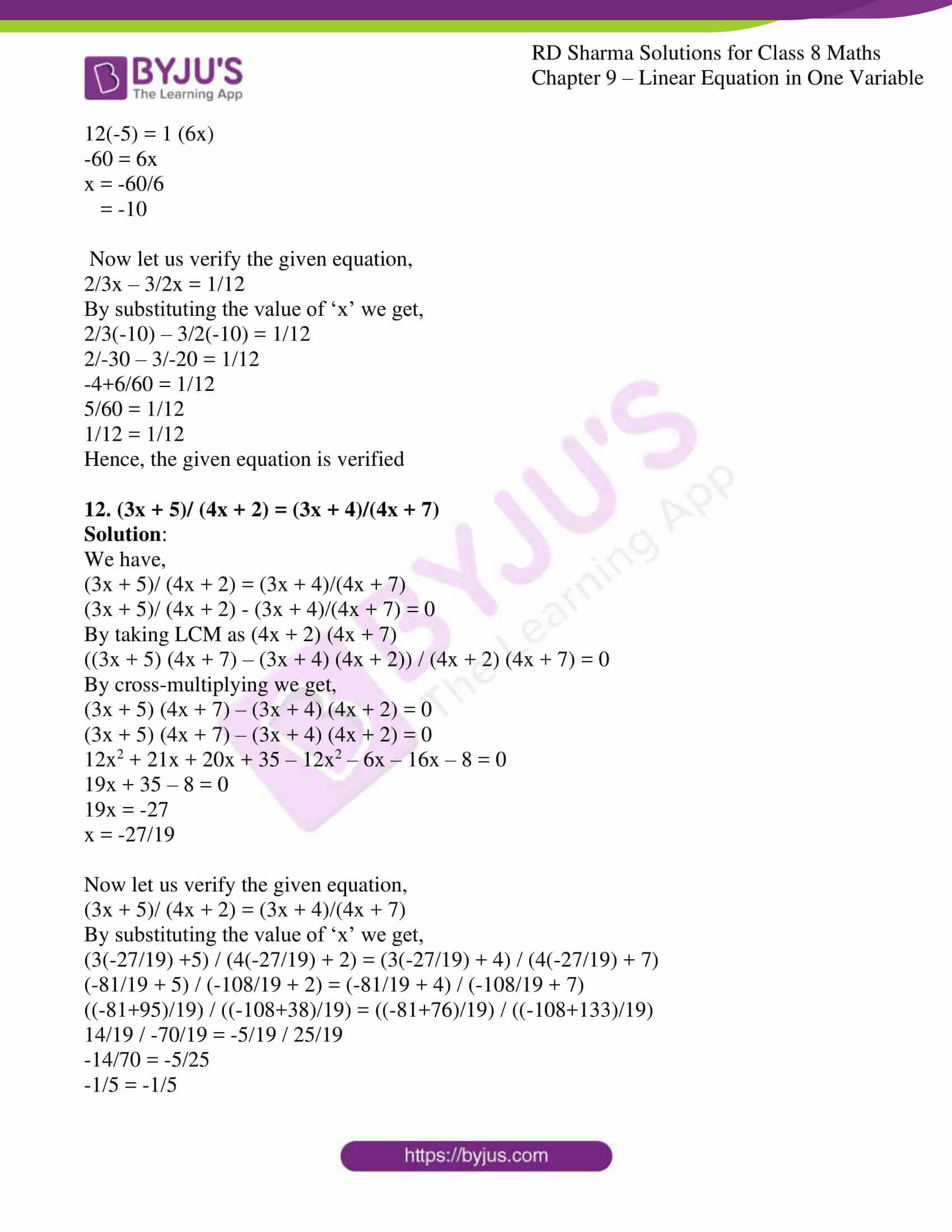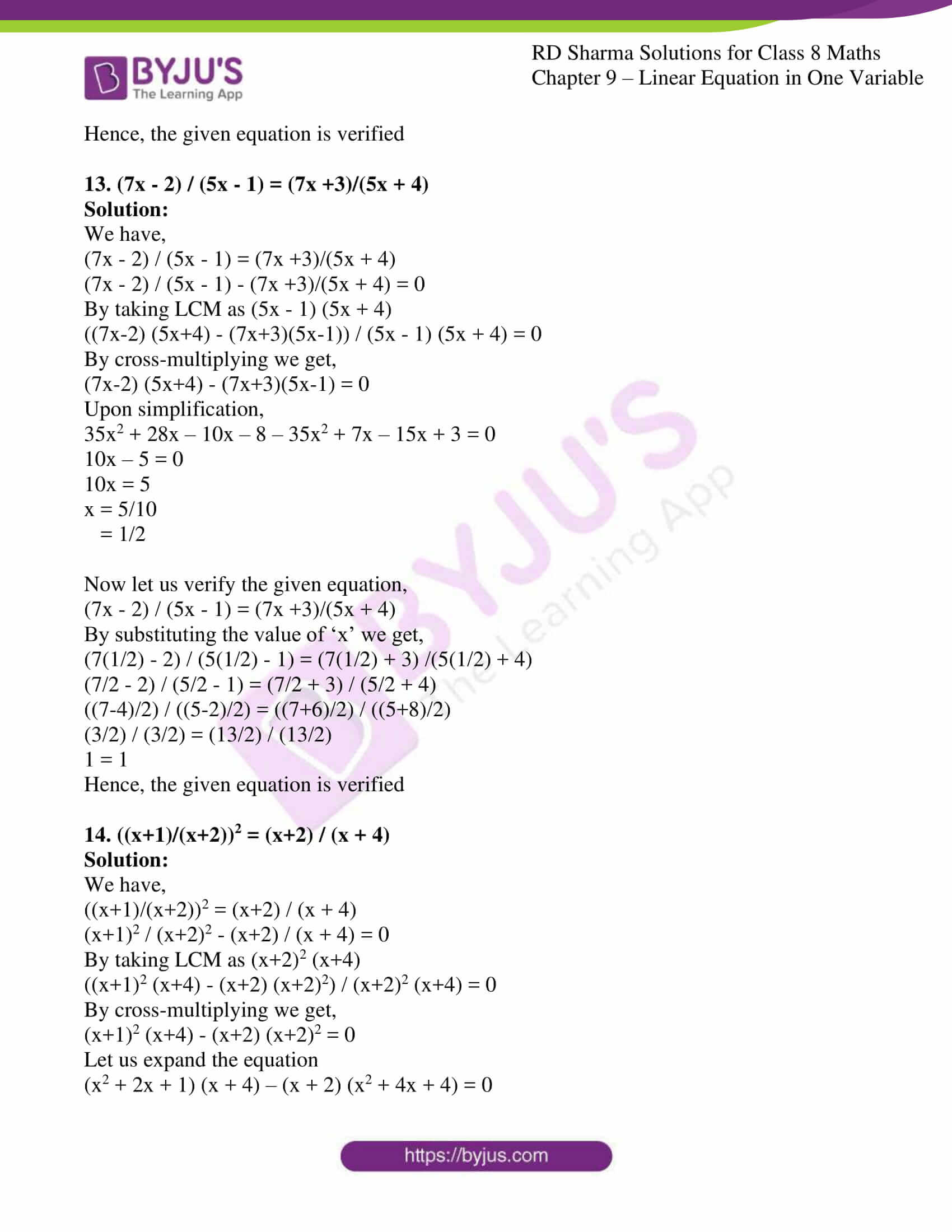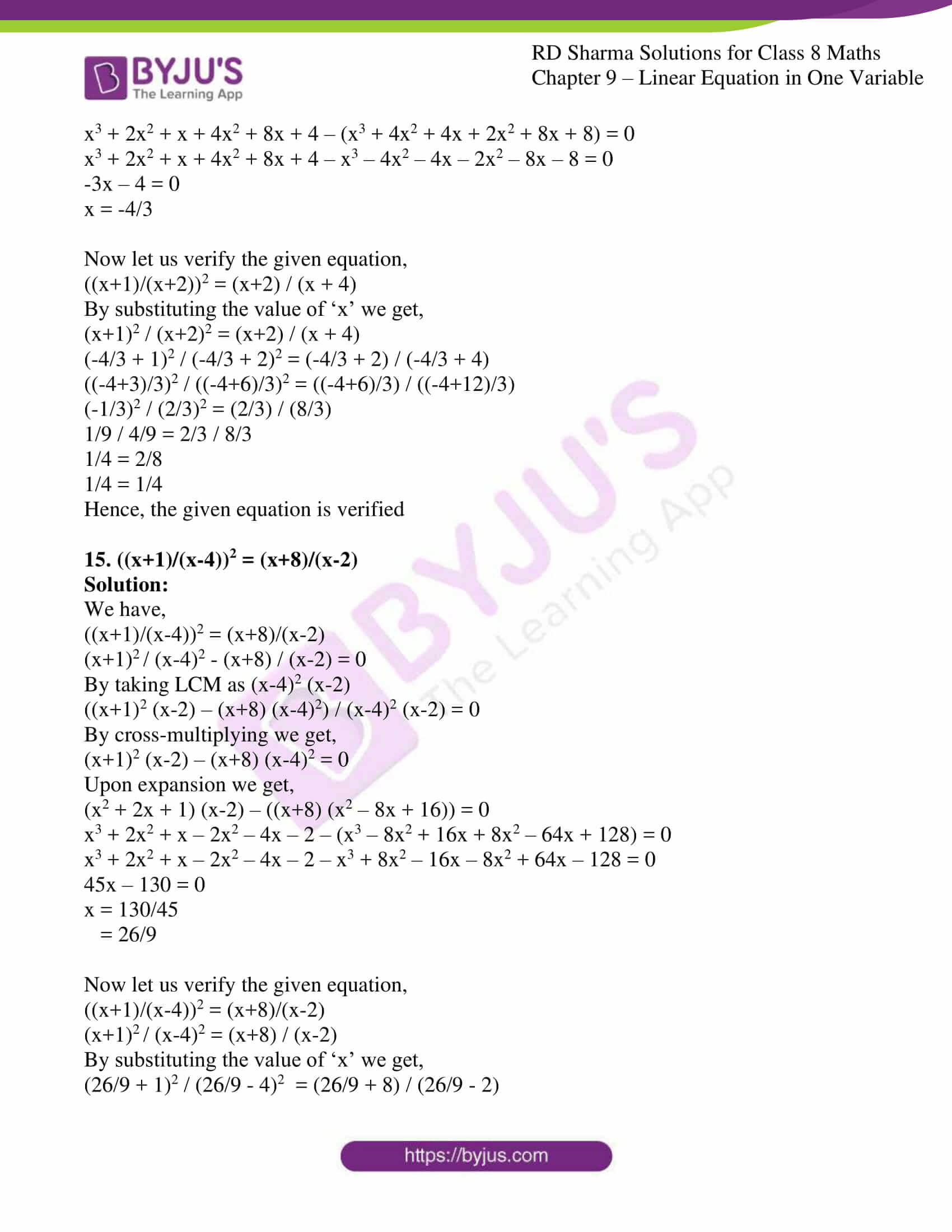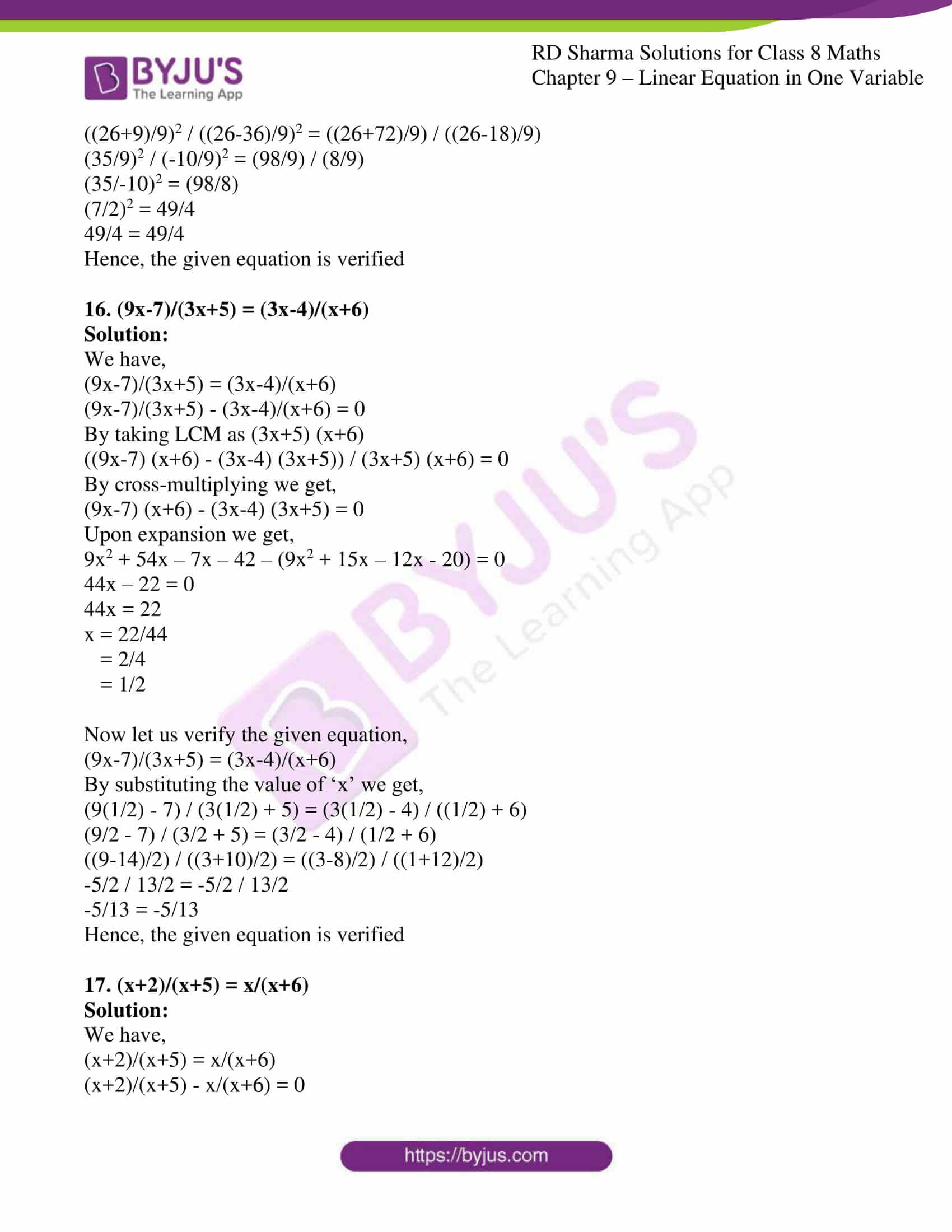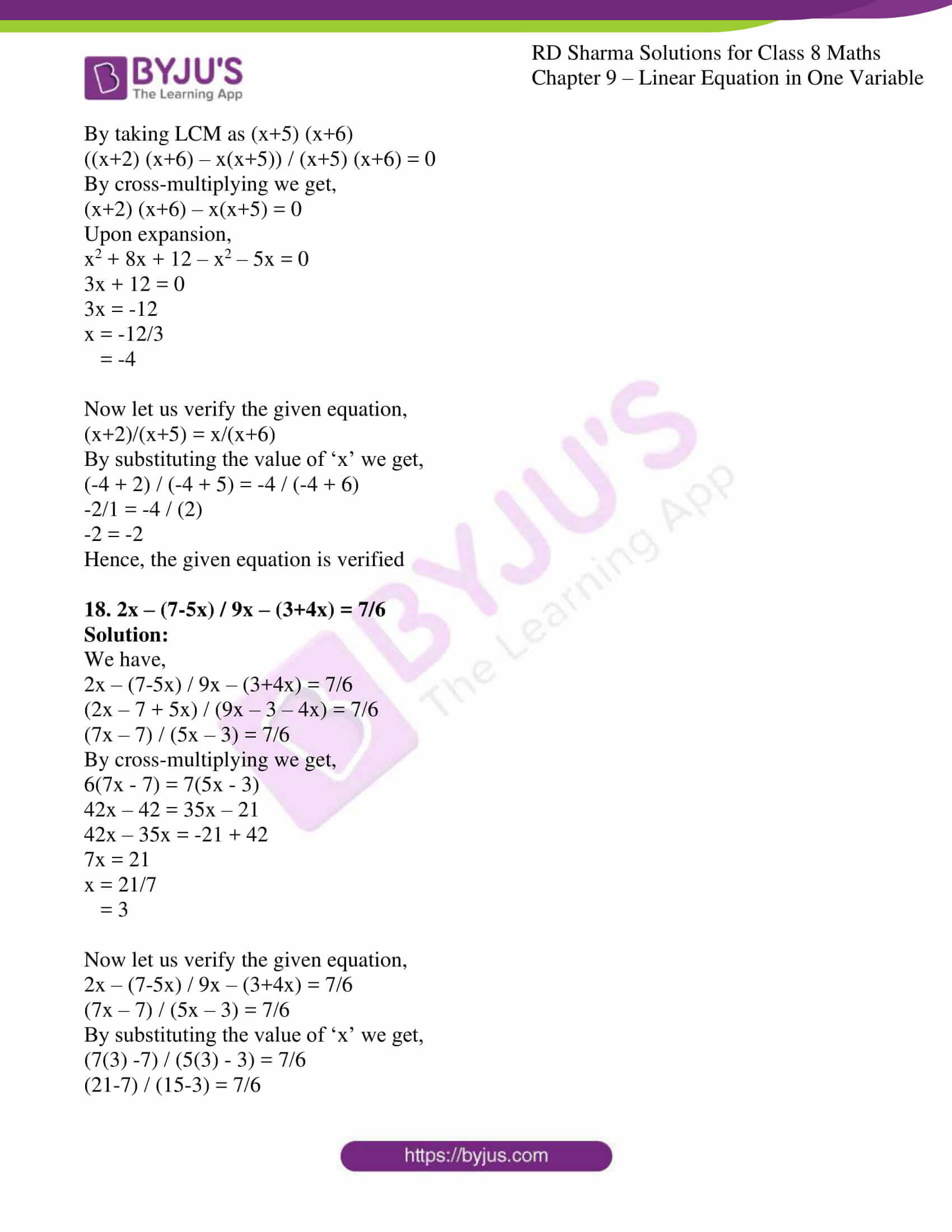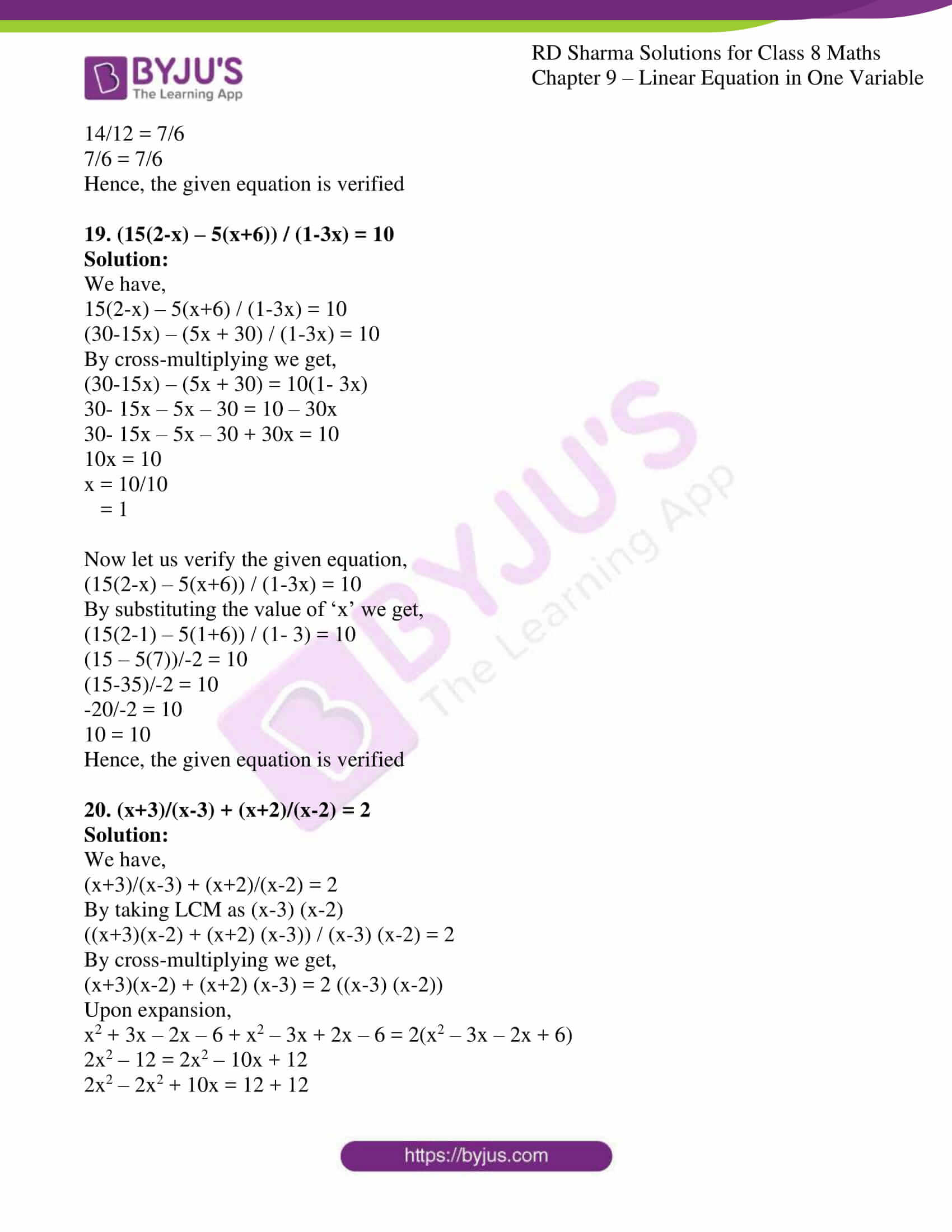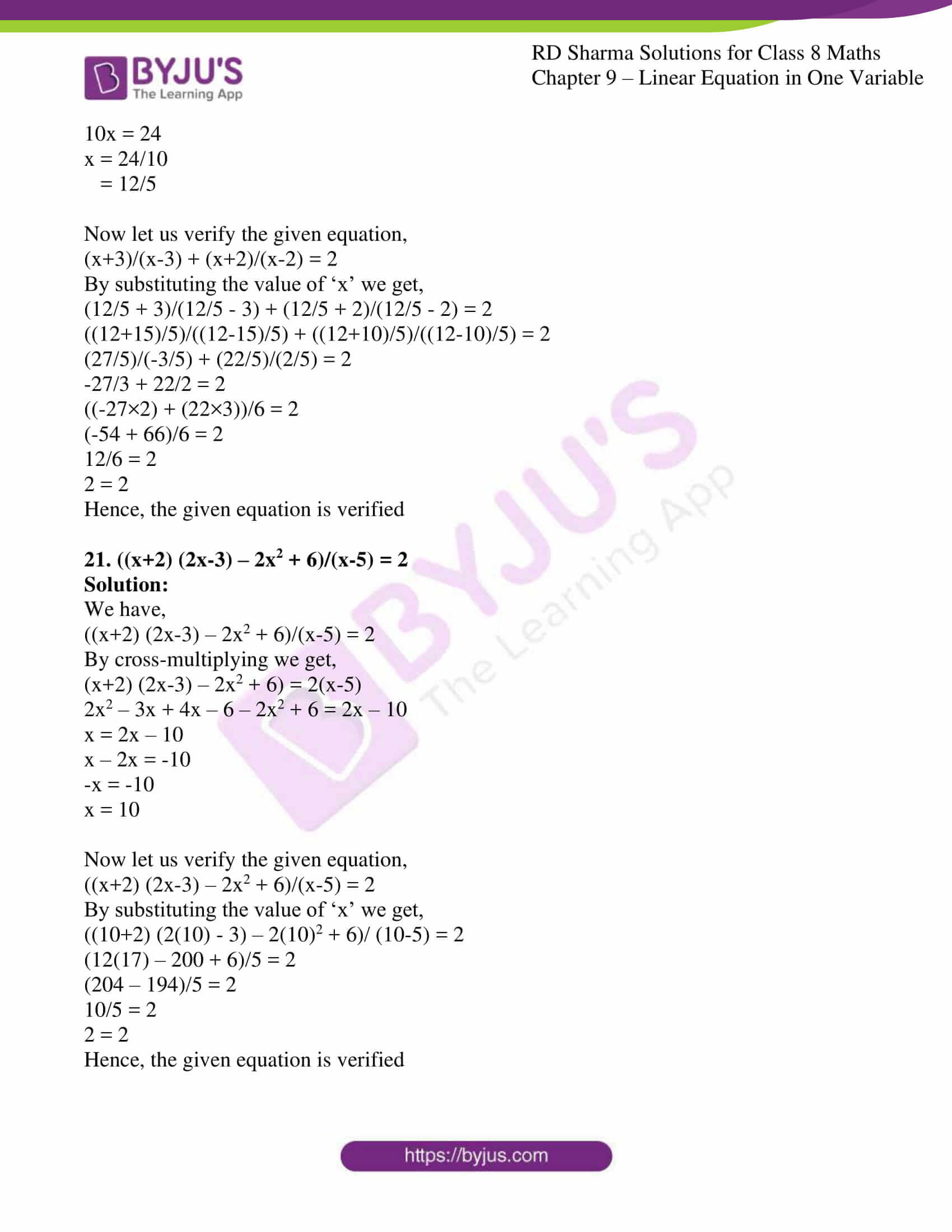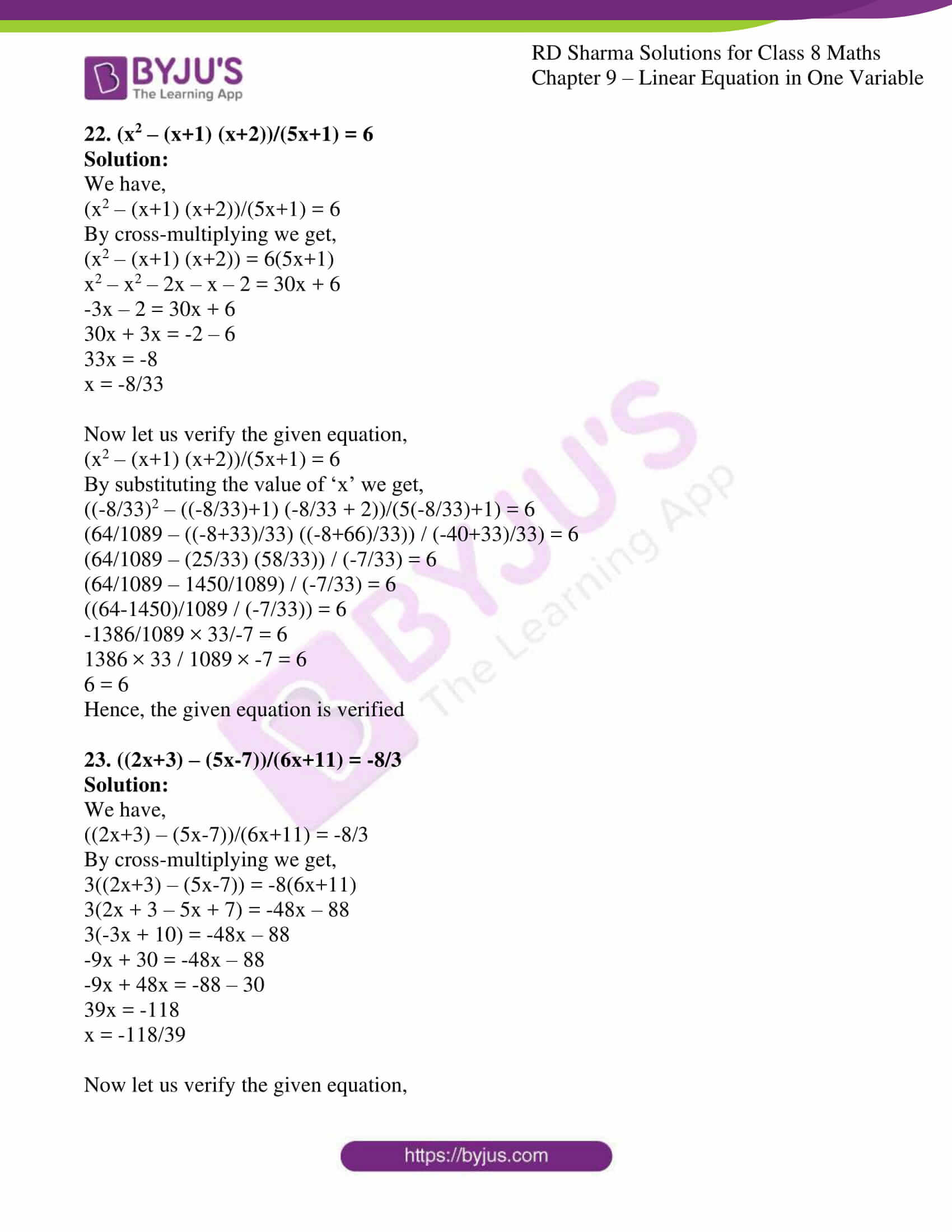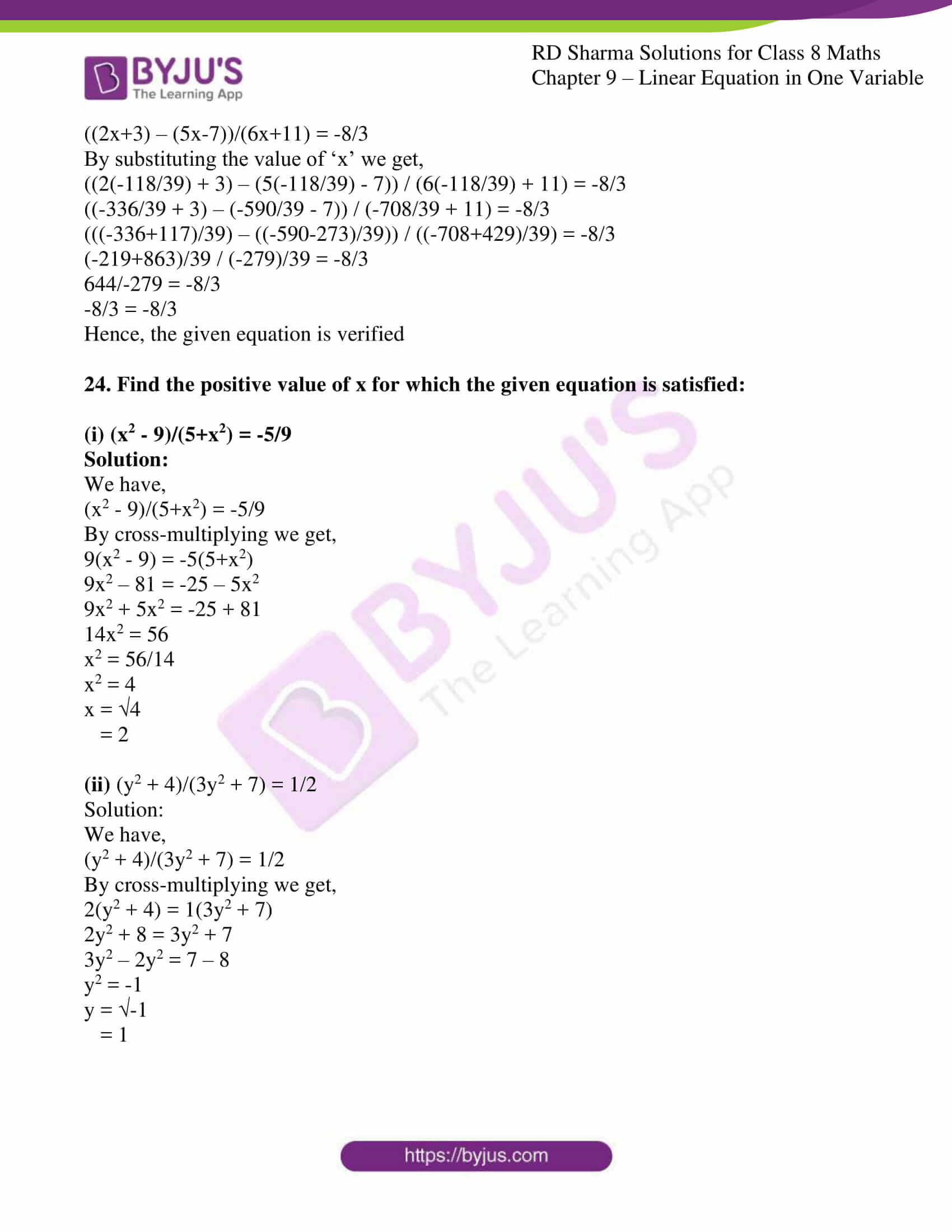### Access Answers to RD Sharma Solutions for Class 8 Maths Exercise 9.3 Chapter 9 Linear Equation in One Variable

1. (2x-3) / (3x+2) = -2/3

Solution:

We have,

(2x-3) / (3x+2) = -2/3

Let us perform cross-multiplication we get,

3(2x – 3) = -2(3x + 2)

6x – 9 = -6x – 4

When rearranged,

6x + 6x = 9 – 4

12x = 5

x = 5/12

Now let us verify the given equation,

(2x-3) / (3x+2) = -2/3

By substituting the value of ‘x’ we get,

(2(5/12) – 3) / (3(5/12) + 2) = -2/3

((5/6)-3) / ((5/4) + 2) = -2/3

((5-18)/6) / ((5+8)/4) = -2/3

(-13/6) / (13/4) = -2/3

(-13/6) × (4/13) = -2/3

-4/6 = -2/3

-2/3 = -2/3

Hence, the given equation is verified

2. (2-y) / (y+7) = 3/5

Solution:

We have,

(2-y) / (y+7) = 3/5

Let us perform cross-multiplication we get,

5(2-y) = 3(y+7)

10 – 5y = 3y + 21

When rearranged,

10 – 21 = 3y + 5y

8y = – 11

y = -11/8

Now let us verify the given equation,

(2-y) / (y+7) = 3/5

By substituting the value of ‘x’ we get,

(2 – (-11/8)) / ((-11/8) + 7) = 3/5

((16+11)/8) / ((-11+56)/8) = 3/5

(27/8) / (45/8) = 3/5

(27/8) × (8/45) = 3/5

27/45 = 3/5

3/5 = 3/5

Hence, the given equation is verified

3. (5x – 7) / (3x) = 2

Solution:

We have,

(5x – 7) / (3x) = 2

Let us perform cross-multiplication we get,

5x – 7 = 2(3x)

5x – 7 = 6x

5x – 6x = 7

-x = 7

x = -7

Now let us verify the given equation,

(5x – 7) / (3x) = 2

By substituting the value of ‘x’ we get,

(5(-7) – 7) / (3(-7)) = 2

(-35 – 7) / -21 = 2

-42/-21 = 2

2 = 2

Hence, the given equation is verified

4. (3x+5) / (2x + 7) = 4

Solution:

We have,

(3x+5) / (2x + 7) = 4

Let us perform cross-multiplication we get,

3x + 5 = 4(2x+7)

3x + 5 = 8x + 28

3x – 8x = 28 – 5

-5x = 23

x = -23/5

Now let us verify the given equation,

(3x+5) / (2x + 7) = 4

By substituting the value of ‘x’ we get,

(3(-23/5) + 5) / (2(-23/5) + 7) = 4

(-69/5 + 5) / (-46/5 + 7) = 4

(-69+25)/5 / (-46+35)/5 = 4

-44/5 / -11/5 = 4

-44/5 × 5/-11 = 4

44/11 = 4

4 = 4

Hence, the given equation is verified

5. (2y + 5) / (y + 4) = 1

Solution:

We have,

(2y + 5) / (y + 4) = 1

Let us perform cross-multiplication we get,

2y + 5 = y + 4

2y – y = 4 – 5

y = -1

Now let us verify the given equation,

(2y + 5) / (y + 4) = 1

By substituting the value of ‘y’ we get,

(2(-1) + 5) / (-1 + 4) = 1

(-2+5) / 3 = 1

3/3 = 1

1 = 1

Hence, the given equation is verified

6. (2x + 1) / (3x – 2) = 5/9

Solution:

We have,

(2x + 1) / (3x – 2) = 5/9

Let us perform cross-multiplication we get,

9(2x + 1) = 5(3x – 2)

18x + 9 = 15x – 10

18x – 15x = -10 – 9

3x = -19

x = -19/3

Now let us verify the given equation,

(2x + 1) / (3x – 2) = 5/9

By substituting the value of ‘x’ we get,

(2(-19/3) + 1) / (3(-19/3) – 2) = 5/9

(-38/3 + 1) / (-57/3 – 2) = 5/9

(-38 + 3)/3 / (-57 – 6)/3 = 5/9

-35/3 / -63/3 = 5/9

-35/3 × 3/-63 = 5/9

-35/-63 = 5/9

5/9 = 5/9

Hence, the given equation is verified

7. (1 – 9y) / (19 – 3y) = 5/8

Solution:

We have,

(1 – 9y) / (19 – 3y) = 5/8

Let us perform cross-multiplication we get,

8(1- 9y) = 5(19-3y)

8 – 72y = 95 – 15y

8 – 95 = 72y – 15y

57y = -87

y = -87/57

= -29/19

Now let us verify the given equation,

(1 – 9y) / (19 – 3y) = 5/8

By substituting the value of ‘y’ we get,

(1 – 9(-29/19)) / (19 – 3(-29/19)) = 5/8

(19+261)/19 / (361+87)/19 = 5/8

280/19 × 19/448 = 5/8

280/ 448 = 5/8

5/8 = 5/8

Hence, the given equation is verified

8. 2x / (3x + 1) = 1

Solution:

We have,

2x / (3x + 1) = 1

Let us perform cross-multiplication we get,

2x = 1(3x + 1)

2x = 3x + 1

2x – 3x = 1

-x = 1

x = -1

Now let us verify the given equation,

2x / (3x + 1) = 1

By substituting the value of ‘x’ we get,

2(-1) / (3(-1) + 1) = 1

-2 /(-3+1) = 1

-2/-2 = 1

1 = 1

Hence, the given equation is verified

9. y – (7 – 8y)/9y – (3 + 4y) = 2/3

Solution:

We have,

y – (7 – 8y)/9y – (3 + 4y) = 2/3

(y – 7 + 8y) / (9y – 3 – 4y) = 2/3

(-7 + 9y) / (5y – 3) = 2/3

Let us perform cross-multiplication we get,

3(-7 + 9y) = 2(5y – 3)

-21 + 27y = 10y – 6

27y – 10y = 21 – 6

17y = 15

y = 15/17

Now let us verify the given equation,

y – (7 – 8y)/9y – (3 + 4y) = 2/3

By substituting the value of ‘y’ we get,

15/17 – (7-8(15/17))/ 9(15/17) – (3 + 4(15/17)) = 2/3

15/17 – (7 – 120/17) / 135/17 – (3 + 60/17) = 2/3

15/17 – ((119-120)/17) / 135/17 – ((51+60)/17) = 2/3

15/17 – (-1/17) / 135/17 – (111/17) = 2/23

((15 + 1)/17) / ((135-111)/17) = 2/3

16/17 / 24/17 = 2/3

16/24 = 2/3

2/3 = 2/3

Hence, the given equation is verified

10. 6/ 2x – (3 – 4x) = 2/3

Solution:

We have,

6/ 2x – (3 – 4x) = 2/3

6/(2x – 3 + 4x) = 2/3

6/(6x – 3) = 2/3

Let us perform cross-multiplication we get,

3(6) = 2(6x – 3)

18 = 12x – 6

12x = 18 + 6

12x = 24

x = 24/12

= 2

Now let us verify the given equation,

6/ 2x – (3 – 4x) = 2/3

6/(6x – 3) = 2/3

By substituting the value of ‘x’ we get,

6/ (6(2) – 3) = 2/3

6/(12-3) = 2/3

6/9 = 2/3

2/3 = 2/3

Hence, the given equation is verified

11. 2/3x – 3/2x = 1/12

Solution:

We have,

2/3x – 3/2x = 1/12

By taking LCM for 2 and 3 which is 6

4-9/6x = 1/12

-5/6x = 1/12

By cross-multiplying we get,

12(-5) = 1 (6x)

-60 = 6x

x = -60/6

= -10

Now let us verify the given equation,

2/3x – 3/2x = 1/12

By substituting the value of ‘x’ we get,

2/3(-10) – 3/2(-10) = 1/12

2/-30 – 3/-20 = 1/12

-4+6/60 = 1/12

5/60 = 1/12

1/12 = 1/12

Hence, the given equation is verified

12. (3x + 5)/ (4x + 2) = (3x + 4)/(4x + 7)

Solution:

We have,

(3x + 5)/ (4x + 2) = (3x + 4)/(4x + 7)

(3x + 5)/ (4x + 2) – (3x + 4)/(4x + 7) = 0

By taking LCM as (4x + 2) (4x + 7)

((3x + 5) (4x + 7) – (3x + 4) (4x + 2)) / (4x + 2) (4x + 7) = 0

By cross-multiplying we get,

(3x + 5) (4x + 7) – (3x + 4) (4x + 2) = 0

(3x + 5) (4x + 7) – (3x + 4) (4x + 2) = 0

12x2 + 21x + 20x + 35 – 12x2 – 6x – 16x – 8 = 0

19x + 35 – 8 = 0

19x = -27

x = -27/19

Now let us verify the given equation,

(3x + 5)/ (4x + 2) = (3x + 4)/(4x + 7)

By substituting the value of ‘x’ we get,

(3(-27/19) +5) / (4(-27/19) + 2) = (3(-27/19) + 4) / (4(-27/19) + 7)

(-81/19 + 5) / (-108/19 + 2) = (-81/19 + 4) / (-108/19 + 7)

((-81+95)/19) / ((-108+38)/19) = ((-81+76)/19) / ((-108+133)/19)

14/19 / -70/19 = -5/19 / 25/19

-14/70 = -5/25

-1/5 = -1/5

Hence, the given equation is verified

13. (7x – 2) / (5x – 1) = (7x +3)/(5x + 4)

Solution:

We have,

(7x – 2) / (5x – 1) = (7x +3)/(5x + 4)

(7x – 2) / (5x – 1) – (7x +3)/(5x + 4) = 0

By taking LCM as (5x – 1) (5x + 4)

((7x-2) (5x+4) – (7x+3)(5x-1)) / (5x – 1) (5x + 4) = 0

By cross-multiplying we get,

(7x-2) (5x+4) – (7x+3)(5x-1) = 0

Upon simplification,

35x2 + 28x – 10x – 8 – 35x2 + 7x – 15x + 3 = 0

10x – 5 = 0

10x = 5

x = 5/10

= 1/2

Now let us verify the given equation,

(7x – 2) / (5x – 1) = (7x +3)/(5x + 4)

By substituting the value of ‘x’ we get,

(7(1/2) – 2) / (5(1/2) – 1) = (7(1/2) + 3) /(5(1/2) + 4)

(7/2 – 2) / (5/2 – 1) = (7/2 + 3) / (5/2 + 4)

((7-4)/2) / ((5-2)/2) = ((7+6)/2) / ((5+8)/2)

(3/2) / (3/2) = (13/2) / (13/2)

1 = 1

Hence, the given equation is verified

14. ((x+1)/(x+2))2 = (x+2) / (x + 4)

Solution:

We have,

((x+1)/(x+2))2 = (x+2) / (x + 4)

(x+1)2 / (x+2)2 – (x+2) / (x + 4) = 0

By taking LCM as (x+2)2 (x+4)

((x+1)2 (x+4) – (x+2) (x+2)2) / (x+2)2 (x+4) = 0

By cross-multiplying we get,

(x+1)2 (x+4) – (x+2) (x+2)2 = 0

Let us expand the equation

(x2 + 2x + 1) (x + 4) – (x + 2) (x2 + 4x + 4) = 0

x3 + 2x2 + x + 4x2 + 8x + 4 – (x3 + 4x2 + 4x + 2x2 + 8x + 8) = 0

x3 + 2x2 + x + 4x2 + 8x + 4 – x3 – 4x2 – 4x – 2x2 – 8x – 8 = 0

-3x – 4 = 0

x = -4/3

Now let us verify the given equation,

((x+1)/(x+2))2 = (x+2) / (x + 4)

By substituting the value of ‘x’ we get,

(x+1)2 / (x+2)2 = (x+2) / (x + 4)

(-4/3 + 1)2 / (-4/3 + 2)2 = (-4/3 + 2) / (-4/3 + 4)

((-4+3)/3)2 / ((-4+6)/3)2 = ((-4+6)/3) / ((-4+12)/3)

(-1/3)2 / (2/3)2 = (2/3) / (8/3)

1/9 / 4/9 = 2/3 / 8/3

1/4 = 2/8

1/4 = 1/4

Hence, the given equation is verified

15. ((x+1)/(x-4))2 = (x+8)/(x-2)

Solution:

We have,

((x+1)/(x-4))2 = (x+8)/(x-2)

(x+1)2 / (x-4)2 – (x+8) / (x-2) = 0

By taking LCM as (x-4)2 (x-2)

((x+1)2 (x-2) – (x+8) (x-4)2) / (x-4)2 (x-2) = 0

By cross-multiplying we get,

(x+1)2 (x-2) – (x+8) (x-4)2 = 0

Upon expansion we get,

(x2 + 2x + 1) (x-2) – ((x+8) (x2 – 8x + 16)) = 0

x3 + 2x2 + x – 2x2 – 4x – 2 – (x3 – 8x2 + 16x + 8x2 – 64x + 128) = 0

x3 + 2x2 + x – 2x2 – 4x – 2 – x3 + 8x2 – 16x – 8x2 + 64x – 128 = 0

45x – 130 = 0

x = 130/45

= 26/9

Now let us verify the given equation,

((x+1)/(x-4))2 = (x+8)/(x-2)

(x+1)2 / (x-4)2 = (x+8) / (x-2)

By substituting the value of ‘x’ we get,

(26/9 + 1)2 / (26/9 – 4)2 = (26/9 + 8) / (26/9 – 2)

((26+9)/9)2 / ((26-36)/9)2 = ((26+72)/9) / ((26-18)/9)

(35/9)2 / (-10/9)2 = (98/9) / (8/9)

(35/-10)2 = (98/8)

(7/2)2 = 49/4

49/4 = 49/4

Hence, the given equation is verified

16. (9x-7)/(3x+5) = (3x-4)/(x+6)

Solution:

We have,

(9x-7)/(3x+5) = (3x-4)/(x+6)

(9x-7)/(3x+5) – (3x-4)/(x+6) = 0

By taking LCM as (3x+5) (x+6)

((9x-7) (x+6) – (3x-4) (3x+5)) / (3x+5) (x+6) = 0

By cross-multiplying we get,

(9x-7) (x+6) – (3x-4) (3x+5) = 0

Upon expansion we get,

9x2 + 54x – 7x – 42 – (9x2 + 15x – 12x – 20) = 0

44x – 22 = 0

44x = 22

x = 22/44

= 2/4

= 1/2

Now let us verify the given equation,

(9x-7)/(3x+5) = (3x-4)/(x+6)

By substituting the value of ‘x’ we get,

(9(1/2) – 7) / (3(1/2) + 5) = (3(1/2) – 4) / ((1/2) + 6)

(9/2 – 7) / (3/2 + 5) = (3/2 – 4) / (1/2 + 6)

((9-14)/2) / ((3+10)/2) = ((3-8)/2) / ((1+12)/2)

-5/2 / 13/2 = -5/2 / 13/2

-5/13 = -5/13

Hence, the given equation is verified

17. (x+2)/(x+5) = x/(x+6)

Solution:

We have,

(x+2)/(x+5) = x/(x+6)

(x+2)/(x+5) – x/(x+6) = 0

By taking LCM as (x+5) (x+6)

((x+2) (x+6) – x(x+5)) / (x+5) (x+6) = 0

By cross-multiplying we get,

(x+2) (x+6) – x(x+5) = 0

Upon expansion,

x2 + 8x + 12 – x2 – 5x = 0

3x + 12 = 0

3x = -12

x = -12/3

= -4

Now let us verify the given equation,

(x+2)/(x+5) = x/(x+6)

By substituting the value of ‘x’ we get,

(-4 + 2) / (-4 + 5) = -4 / (-4 + 6)

-2/1 = -4 / (2)

-2 = -2

Hence, the given equation is verified

18. 2x – (7-5x) / 9x – (3+4x) = 7/6

Solution:

We have,

2x – (7-5x) / 9x – (3+4x) = 7/6

(2x – 7 + 5x) / (9x – 3 – 4x) = 7/6

(7x – 7) / (5x – 3) = 7/6

By cross-multiplying we get,

6(7x – 7) = 7(5x – 3)

42x – 42 = 35x – 21

42x – 35x = -21 + 42

7x = 21

x = 21/7

= 3

Now let us verify the given equation,

2x – (7-5x) / 9x – (3+4x) = 7/6

(7x – 7) / (5x – 3) = 7/6

By substituting the value of ‘x’ we get,

(7(3) -7) / (5(3) – 3) = 7/6

(21-7) / (15-3) = 7/6

14/12 = 7/6

7/6 = 7/6

Hence, the given equation is verified

19. (15(2-x) – 5(x+6)) / (1-3x) = 10

Solution:

We have,

15(2-x) – 5(x+6) / (1-3x) = 10

(30-15x) – (5x + 30) / (1-3x) = 10

By cross-multiplying we get,

(30-15x) – (5x + 30) = 10(1- 3x)

30- 15x – 5x – 30 = 10 – 30x

30- 15x – 5x – 30 + 30x = 10

10x = 10

x = 10/10

= 1

Now let us verify the given equation,

(15(2-x) – 5(x+6)) / (1-3x) = 10

By substituting the value of ‘x’ we get,

(15(2-1) – 5(1+6)) / (1- 3) = 10

(15 – 5(7))/-2 = 10

(15-35)/-2 = 10

-20/-2 = 10

10 = 10

Hence, the given equation is verified

20. (x+3)/(x-3) + (x+2)/(x-2) = 2

Solution:

We have,

(x+3)/(x-3) + (x+2)/(x-2) = 2

By taking LCM as (x-3) (x-2)

((x+3)(x-2) + (x+2) (x-3)) / (x-3) (x-2) = 2

By cross-multiplying we get,

(x+3)(x-2) + (x+2) (x-3) = 2 ((x-3) (x-2))

Upon expansion,

x2 + 3x – 2x – 6 + x2 – 3x + 2x – 6 = 2(x2 – 3x – 2x + 6)

2x2 – 12 = 2x2 – 10x + 12

2x2 – 2x2 + 10x = 12 + 12

10x = 24

x = 24/10

= 12/5

Now let us verify the given equation,

(x+3)/(x-3) + (x+2)/(x-2) = 2

By substituting the value of ‘x’ we get,

(12/5 + 3)/(12/5 – 3) + (12/5 + 2)/(12/5 – 2) = 2

((12+15)/5)/((12-15)/5) + ((12+10)/5)/((12-10)/5) = 2

(27/5)/(-3/5) + (22/5)/(2/5) = 2

-27/3 + 22/2 = 2

((-27×2) + (22×3))/6 = 2

(-54 + 66)/6 = 2

12/6 = 2

2 = 2

Hence, the given equation is verified

21. ((x+2) (2x-3) – 2x2 + 6)/(x-5) = 2

Solution:

We have,

((x+2) (2x-3) – 2x2 + 6)/(x-5) = 2

By cross-multiplying we get,

(x+2) (2x-3) – 2x2 + 6) = 2(x-5)

2x2 – 3x + 4x – 6 – 2x2 + 6 = 2x – 10

x = 2x – 10

x – 2x = -10

-x = -10

x = 10

Now let us verify the given equation,

((x+2) (2x-3) – 2x2 + 6)/(x-5) = 2

By substituting the value of ‘x’ we get,

((10+2) (2(10) – 3) – 2(10)2 + 6)/ (10-5) = 2

(12(17) – 200 + 6)/5 = 2

(204 – 194)/5 = 2

10/5 = 2

2 = 2

Hence, the given equation is verified

22. (x2 – (x+1) (x+2))/(5x+1) = 6

Solution:

We have,

(x2 – (x+1) (x+2))/(5x+1) = 6

By cross-multiplying we get,

(x2 – (x+1) (x+2)) = 6(5x+1)

x2 – x2 – 2x – x – 2 = 30x + 6

-3x – 2 = 30x + 6

30x + 3x = -2 – 6

33x = -8

x = -8/33

Now let us verify the given equation,

(x2 – (x+1) (x+2))/(5x+1) = 6

By substituting the value of ‘x’ we get,

((-8/33)2 – ((-8/33)+1) (-8/33 + 2))/(5(-8/33)+1) = 6

(64/1089 – ((-8+33)/33) ((-8+66)/33)) / (-40+33)/33) = 6

(64/1089 – (25/33) (58/33)) / (-7/33) = 6

(64/1089 – 1450/1089) / (-7/33) = 6

((64-1450)/1089 / (-7/33)) = 6

-1386/1089 × 33/-7 = 6

1386 × 33 / 1089 × -7 = 6

6 = 6

Hence, the given equation is verified

23. ((2x+3) – (5x-7))/(6x+11) = -8/3

Solution:

We have,

((2x+3) – (5x-7))/(6x+11) = -8/3

By cross-multiplying we get,

3((2x+3) – (5x-7)) = -8(6x+11)

3(2x + 3 – 5x + 7) = -48x – 88

3(-3x + 10) = -48x – 88

-9x + 30 = -48x – 88

-9x + 48x = -88 – 30

39x = -118

x = -118/39

Now let us verify the given equation,

((2x+3) – (5x-7))/(6x+11) = -8/3

By substituting the value of ‘x’ we get,

((2(-118/39) + 3) – (5(-118/39) – 7)) / (6(-118/39) + 11) = -8/3

((-336/39 + 3) – (-590/39 – 7)) / (-708/39 + 11) = -8/3

(((-336+117)/39) – ((-590-273)/39)) / ((-708+429)/39) = -8/3

(-219+863)/39 / (-279)/39 = -8/3

644/-279 = -8/3

-8/3 = -8/3

Hence, the given equation is verified

24. Find the positive value of x for which the given equation is satisfied:

(i) (x2 – 9)/(5+x2) = -5/9

Solution:

We have,

(x2 – 9)/(5+x2) = -5/9

By cross-multiplying we get,

9(x2 – 9) = -5(5+x2)

9x2 – 81 = -25 – 5x2

9x2 + 5x2 = -25 + 81

14x2 = 56

x2 = 56/14

x2 = 4

x = √4

= 2

(ii) (y2 + 4)/(3y2 + 7) = 1/2

Solution:

We have,

(y2 + 4)/(3y2 + 7) = 1/2

By cross-multiplying we get,

2(y2 + 4) = 1(3y2 + 7)

2y2 + 8 = 3y2 + 7

3y2 – 2y2 = 7 – 8

y2 = -1

y = √-1

= 1

#### 1 Comment

1. Jagmal Dahiya

that is a good maths for students